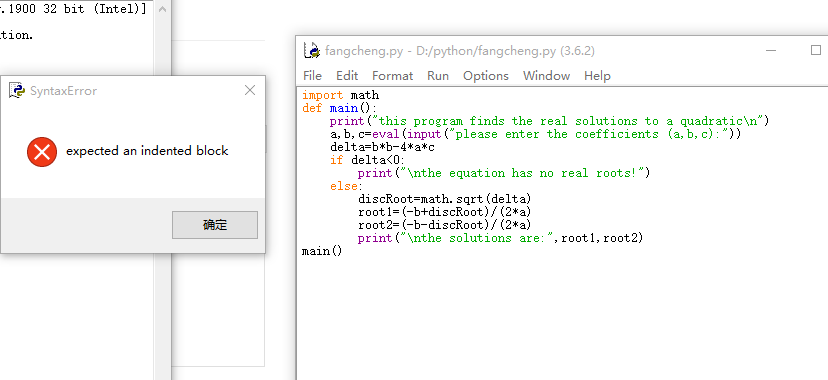python解二分方程不知道哪里出错`````` import math

def main():

print("this program finds the real solutions to a quadratic\n")

delta=b*b-4*a*c

if delta<0:

print("\nthe equation has no real roots!")
else:

discRoot=math.sqrt(delta)

root1=(-b+discRoot)/(2*a)

root2=(-b-discRoot)/(2*a)

print("\nthe solutions are:",root1,root2)
main()
``````

2个回答

``````a,b,c=eval(input("a,b,c:"))
if  a==0:
if  b==0:
if c==0:
print("x为任意值")
else:
print("无解")
else:
print("x=",-1*c/b)      # bx + c =0
else:
dt=b*b-4*a*c
if dt==0:
print("x1=x2=",-1*b/2/a)
elif dt >0:
print("x1=",(-b+dt**0.5)/(2*a),",x2=",(-b-dt**0.5)/(2*a))
else:
print("x1=", -1 * b / 2 / a, "+", (-1 * dt) ** 0.5 / (2 * a), "i")
print("x2=", -1 * b / 2 / a, "-", (-1 * dt) ** 0.5 / (2 * a), "i")
``````